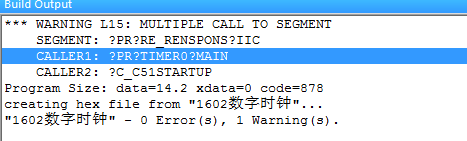//main.c//
#include
#include"IIC.H"
#include
#define uchar unsigned char
uchar count,keynum;
char sec,min,hour;

sbit lcden=P1^2; //液晶使能端
sbit lcdrs=P2^0;
sbit buzz=P0^6;
sbit key1=P3^0;
sbit key2=P3^1;
sbit key3=P3^2;
uchar code table[]="2017-06-07";
void Delay5ms() //@11.0592MHz
{
unsigned char i, j;

``````i = 54;
j = 199;
do
{
while (--j);
} while (--i);
``````

}

void di()
{
P2=0XA0;
buzz=1;
Delay5ms();
P2=0x00;
}
void write_com(unsigned char date)
{
lcdrs=0;
lcden=1;
P0=date;
Delay5ms();

lcden=0;
}

void write_data(unsigned char date)
{
lcdrs=1;
lcden=1;
P0=date;
Delay5ms();
lcden=0;
}
{
uchar shi,ge;
shi=date/10;
ge=date%10;
write_data(0x30+ge);
write_data(0x30+shi);
}
void init1()
{
uchar num;
sec=0;
min=0;
hour=0;
keynum=0;
count=0;
init_24c02();
write_com(0x38); //模式
write_com(0x0c); //开显示,显示光标,不闪烁
write_com(0x06); //指针加1
write_com(0x01); //清屏
for(num=0;num<12;num++)
{
write_data(table[num]);
}
TMOD=0X01;
TH0=(65536-50000)/256;
TL0=(65536-50000)%256;
EA=1;
ET0=1;
TR0=1;
write_com(0x80+0x40+6);
write_data(':');
Delay5ms();
write_com(0x80+0x40+9);
write_data(':');
Delay5ms();
}
void key_scan()
{

`````` if(key1)
{
TR0=0;
Delay5ms();
if(key1)
{
while(!key1);
di();
keynum++;
if(keynum==1)
{
write_com(0x80+0x40+10);
write_data(0x0f);

}
if(keynum==2)
{
write_com(0x80+0x40+7);
}
if(keynum==3)
{
write_com(0x80+0x40+4);

}
if(keynum==4)
{
keynum=0;
write_com(0x0c);
TR0=1;
}

}
if(!keynum)
{
if(key2)
{
Delay5ms();
if(key2)
{
while(!key3);
di();
if(keynum==1)
{
sec++;
if(sec==60)
{
sec=0;
}
write_sfm(10,sec);
write_com(0x80+0x40+10);
}
if(keynum==2)
{
min++;
if(min==60)
{
min=0;
}
write_sfm(7,min);
write_com(0x80+0x40+7);
}
if(keynum==3)
{
hour++;
if(hour==60)
{
hour=0;
}
write_sfm(4,hour);
write_com(0x80+0x40+4);
}
}
}
if(key3)
{
Delay5ms();
if(key3)
{
while(!key3);
di();
if(keynum==1)
{
sec--;
if(sec==-1)
{
sec=60;
}
write_sfm(10,sec);
write_com(0x80+0x40+10);
}
if(keynum==2)
{
min--;
if(min==0)
{
min=60;
}
write_sfm(7,min);
write_com(0x80+0x40+7);
}
if(keynum==3)
{
hour--;
if(hour==0)
{
hour=60;
}
write_sfm(4,hour);
write_com(0x80+0x40+4);
}
}
}
}
}
``````

}

void main()
{

init1();
while(1)
{
key_scan();
}
}

void timer0() interrupt 1
{

TH0=(65536-50000)/256;
TL0=(65536-50000)%256;
count++;
if(count==20)
{
count=0;
sec++;
if(sec==60)
{

sec=0;

min++;
if(min==60)
{
min=0;
hour++;
if(hour==60)
{
hour=0;
}
write_sfm(4,hour);
}
write_sfm(7,min);
}
write_sfm(10,sec);
}
}
//IIC.c//
#include
#include "IIC.H"
#include
void delay0()
{ ;;}

void Delay5us()

{
unsigned char i;

``````_nop_();
_nop_();
i = 12;
while (--i);
``````

}

//开始信号
void start()

{
SDA=1;

Delay5us();
SCL=1;
Delay5us();
SDA=0;
Delay5us();
SCL=0;
Delay5us();
}
//停止信号
void stop()

{
SDA=0;
Delay5us();
SCL=1;
Delay5us();
SDA=1;
Delay5us();
}
//主机应答反映
void send_renspons(bit date)

{
SDA=date;
SCL=1;
Delay5us();
SCL=0;
Delay5us();
}
//从机应答信号
bit re_renspons()

{
bit temp;
SDA=1;
Delay5us();
SCL=1;
Delay5us();
if(SDA==1)
temp=0;
else
temp=1;
SCL=0;
Delay5us();
return temp;
}
void init_24c02()
{
SDA=1;
delay0();
SCL=1;
delay0();
}
//写数据_字节
void write_date(unsigned char date)
{ unsigned char i;
for (i=0;i<8;i++)
{
if ((date<<i)&0x80)
SDA=1;
else
SDA=0;
SCL=1;
Delay5us();
SCL=0;
Delay5us();
}

re_renspons();
}
//读数据——字节

{
unsigned char i,temp;
SDA=1;
Delay5us();
for (i=0;i<8;i++)
{
temp<<=1;
SCL=1;
Delay5us();
if(SDA==1)
temp=temp+1;
SCL=0;
Delay5us();

``````            }
return temp;
``````

}
//写一个字节

{
start();
write_date(0xa0);
write_date(date);
stop();
}
//读一个字节

{
unsigned char temp;
start();
write_date(0xa0);
start();
write_date(0xa1);
send_renspons(1);
stop();
return temp;
}
//IIC.H//
#ifndef IIC_H
#define IIC_H

sbit SCL=P2^0;
sbit SDA=P2^1;

void init_24c02();

#endif2个回答AT89C51如何下载程序 用什么软件
atmel公司的AT89C51，注意是AT89C51 老版单片机 如何下载程序 串口的还有usbisp的就不用说了 下载不了 请行家指教
ESP8266与STC89C51单片机通信，为什么单片机可以向ESP8266发送AT指令，但ESP8266发送的数据单片机接收不到？

51单片机之间电信号的相互作用

51单片机显示水平度程序

AT89S52型号的C51单片机是否支持多线程
AT89S52型号的C51单片机是否支持多线程，怎样在函数或者程序中调用？

#include "reg52.h"#include "intrins.h"sbit SDA=P2^1;//总线连接口定义sbit SCL=P2^0;//总线连接口定义sbit duan=P2^2;sbit wei=P2^3;typedef unsigned char uchar;uchar duanma[]={0x3f,0x06,0x5b,0x4f,0x66,0x6d,0x7d,0x07,0x7f,0x6f};uchar weima[]={0xfe,0xfd,0xfb,0xf7,0xef,0xdf,0xbf,0x7f};uchar t;void delay(uchar m)//us延时作用{ while(--m);}void delays(uchar t)//ms延时作用{ while(t--) { delay(245); delay(245); }}/*void display(uchar firstbit,uchar weishu){ static unsigned char i; P0=0; duan=1; duan=0; P0=weima[firstbit+i]; wei=1; wei=0; P0=t[i]; duan=1; duan=0; i++; if(i==weishu) i=0;}*//*void time_0()interrupt 1{ TH0=(65536-2000)/256; TL0=(65536-2000)%256; display(0,8);}*/void iic_start()//开始通知芯片读写开始。{ SDA=1;//上来先让数据线置高电平 SCL=1;//接着让时钟线置高电平 delay(5);//SDA和SCL同时为高电平保持4.7us以上 _nop_();//1.805us,共5.78us,下面SDA=0；是下降沿，不能计算在延时时间中 SDA=0;//在时钟线为高电平，而数据线由高电平到低电平的过程读写芯片开始进行.//下降沿 delay(5);//SDA低电平保持4us以上，这里是4.34us满足要求。 //注意开始后SCL为1，SDA为0； }void iic_inti()//总线初始化{ SCL=1;//=-----————————————————————>>>>>>>>>>>>>让时钟线和数据线都为高电平 SDA=1;//------——————————————————>>>>>>>>>>>>让时钟线和数据线都为高电平 delay(5);}void iic_stop(){ SDA=0; _nop_();//准备状态 SCL=1; //————————————————————————>>>>>>>>结束信号是时钟线为高电平，而数据线由低电平到高电平的过程 delay(5);//该稳态需要保持时间为4us以上。 SDA=1;//SCL高电平期间,SDA来一个上升沿， delay(5);//SDA保持4US以上，4.34加上函数返回时间大于4.7us； //注意结束时SCL和SDA都为1；}void iic_sendbyte(uchar bytedate)//发送一个字节{ uchar i,temp; temp=bytedate; for(i=0;i<8;i++) { temp=temp<<1;//移动后最高位到了PSW寄存器的CY位中。 SCL=0;//准备发送阶段 _nop_();//稳定一下 SDA=CY;//将发送的数据一位位的发送到CY位的SDA上 _nop_();//稳定一下 SCL=1;//每一个高电平期间，IC器件都会将数据取走 _nop_();//稳定一下 } SCL=0;//如果写成SCL=1，SDA=1；就是停止信号了，所以不能那样写 _nop_(); SDA=1;//释放总线，数据总线不用时要释放 _nop_();}uchar iic_readbyte(){ uchar i,temp; SCL=0;//准备读数据 _nop_(); SDA=1;//释放总线 _nop_(); for(i=0;i<8;i++) { SCL=1;//MCU开始取数据 delay(5);//SCL为高电平后，IC器件就会将一位数据送到SDA上， //总共用时不会大于4.34us的,然后就可以让MCU度SDA了 temp=(temp<<1)|SDA;//读一位，保存到temp中。 SCL=0; delay(5); } return temp;}bit iic_checkACK()//处理信号{ uchar errcount=255;//定义超时量为255次 SCL=1; _nop_(); while(SDA) { //在一段时间内检测到SDA=0的话认为是应答信号 if(0==errcount) { SCL=0;//钳住总线 _nop_(); return 0;//没有应答信号 } errcount--; } SCL=0;//钳住总线，为下一次通信做准备 _nop_(); return 1;//成功处理应答信号}void iic_sendACK(bit b_ACK)//发送应答或非应答信号{ SCL=0;//准备 _nop_(); if(b_ACK)//ACK { SDA=0; } else //UACK { SDA=1; } _nop_(); SCL=1; delay(5);//大于4us的延时 SCL=0;// 钳住SCL,以便接受数据. _nop_();}void AT24c02_writebyte(unsigned char addr,unsigned char byte)//向24c02写一个字节数据{ iic_start(); iic_sendbyte(0xa0);//MCU写控制字，前四位固定1010，后三位地址0，末位0是写数据的模式 iic_checkACK();//MCU 处理应答信号 iic_sendbyte(addr);// 准备在指定的地址写入 iic_checkACK(); iic_sendbyte(byte);//写数据 iic_checkACK(); iic_stop(); delays(2);//按字节写入时，24c02在接收到停止信号后，将数据擦写到内部，这需要时间 //并且在这段时间内不会响应总线上的任何请求,固让MCU有2毫秒以上的等待 } void AT24c02_writeData(unsigned char address,unsigned char numbytes,unsigned char *buf)//写入任意长度数据 { while(numbytes--) { AT24c02_writebyte(address++,*buf++); }} void AT24c02_readData(unsigned char beignAddr,unsigned char dataSize,unsigned char *buf)//读取任意长度字节 { iic_start();//起始信号 iic_sendbyte(0xa0);//控制字，写的模式//控制字，读的模式//1010(固定模式)000,地址;最后一位是控制读或写的模式,1为读,0为写 iic_checkACK();//处理应答信号 iic_sendbyte(beignAddr);//发送地址 iic_checkACK();//处理应答信号 iic_start();//发送起始信号 iic_sendbyte(0xa1);//控制字，读的模式//1010(固定模式)000,地址;最后一位是控制读或写的模式,1为读,0为写 iic_checkACK();//处理应答信号 while(dataSize--) { *buf++=iic_readbyte();//读取一个个字节并保存到缓冲区buf中， iic_sendACK(dataSize);//发送应答，当dataSize为0时发送非应答 } iic_stop();//发送停止信号}unsigned char dat[]={0x7f,0xbf,0xdf,0xef, 0xf7,0xfb,0xfd,0xfe, 0xff,0xff,0x00,0x00, 0x55,0x55,0xaa,0xaa };//ram允许情况可以无限添加i;void main(){ uchar i; iic_inti(); AT24c02_writeData(0xae,16,dat); //写入24c02 //第一个数据0xae，代表大地址，第二个1，代表小弟指，一个24c02的小地址的范围是0--250, //第三个是代表数据的地址 delays(1); for(i=0;i<16;i++) dat[i]=0; AT24c02_readData(0xae,16,dat); //从24c02读数据 //第一个数据0xae，代表大地址，第二个1，代表小弟指，一个24c02的小地址的范围是0--250, //第三个是代表数据的地址 while(1) { for(i=0;i<16;i++) //查表 { delays(200); P1=dat[i]; } }} 这个程序为什么只有插上数据线和时钟线才能出现跑马灯效果，不插为什么led灯不亮呢。

51单片机如何做出科学计算器
AT89C51单片机 LCD1602 4*4矩阵键盘 8个独立按键 如何做出科学计算器

《奇巧淫技》系列-python！！每天早上八点自动发送天气预报邮件到QQ邮箱

11月8日，由中国信息通信研究院、中国通信标准化协会、中国互联网协会、可信区块链推进计划联合主办，科技行者协办的2019可信区块链峰会将在北京悠唐皇冠假日酒店开幕。 　　区块链技术被认为是继蒸汽机、电力、互联网之后，下一代颠覆性的核心技术。如果说蒸汽机释放了人类的生产力，电力解决了人类基本的生活需求，互联网彻底改变了信息传递的方式，区块链作为构造信任的技术有重要的价值。 　　1...

C语言魔塔游戏

Android性能优化(4)：UI渲染机制以及优化

Python爬虫爬取淘宝，京东商品信息

Java工作4年来应聘要16K最后没要,细节如下。。。

SpringBoot2.x系列教程（三十六）SpringBoot之Tomcat配置
Spring Boot默认内嵌的Tomcat为Servlet容器，关于Tomcat的所有属性都在ServerProperties配置类中。同时，也可以实现一些接口来自定义内嵌Servlet容器和内嵌Tomcat等的配置。 关于此配置，网络上有大量的资料，但都是基于SpringBoot1.5.x版本，并不适合当前最新版本。本文将带大家了解一下最新版本的使用。 ServerProperties的部分源...
Python绘图，圣诞树，花，爱心 | Turtle篇

CPU对每个程序员来说，是个既熟悉又陌生的东西？ 如果你只知道CPU是中央处理器的话，那可能对你并没有什么用，那么作为程序员的我们，必须要搞懂的就是CPU这家伙是如何运行的，尤其要搞懂它里面的寄存器是怎么一回事，因为这将让你从底层明白程序的运行机制。 随我一起，来好好认识下CPU这货吧 把CPU掰开来看 对于CPU来说，我们首先就要搞明白它是怎么回事，也就是它的内部构造，当然，CPU那么牛的一个东...

2020年全新Java学习路线图，含配套视频，学完即为中级Java程序员！！

B 站上有哪些很好的学习资源?

Web播放器解决了在手机浏览器和PC浏览器上播放音视频数据的问题，让视音频内容可以不依赖用户安装App，就能进行播放以及在社交平台进行传播。在视频业务大数据平台中，播放数据的统计分析非常重要，所以Web播放器在使用过程中，需要对其内部的数据进行收集并上报至服务端，此时，就需要对发生在其内部的一些播放行为进行事件监听。 那么Web播放器事件监听是怎么实现的呢？ 01 监听事件明细表 名...
3万字总结，Mysql优化之精髓

HTML5适合的情人节礼物有纪念日期功能